# Angular Acceleration Formula

Angular Acceleration Formula

The angular acceleration of a rotating object is the rate at which the angular velocity changes with respect to time. It is the change in the angular velocity, divided by the change in time. The average angular acceleration is the change in the angular velocity, divided by the change in time. The angular acceleration is a vector that points in a direction along the rotation axis. The magnitude of the angular acceleration is given by the formula below. The unit of angular acceleration is radians/s2.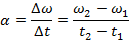Δω = change in angular velocity (radians/s)

Δt = change in time (s)

ω1 = initial angular velocity (radians/s)

t1 = initial time (s)

t2= final time (s)

Angular Acceleration Formula Questions:

1) A disc in a DVD player starts from rest, and then begins spinning when the user presses "Play". After 4.00 s, the disc is spinning at 160 radians/s. What was the average angular acceleration of the disc?

Answer: At the initial time (t1 = 0 s), the angular velocity was ω1 = 0 radians/s. At the final time (t2 = 4.00 s), the angular velocity of the disc was ω1 = 160 radians/s. The average angular acceleration can be found using the formula: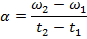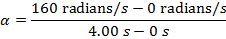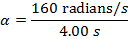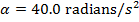Between the initial and final times, the average angular acceleration of the disc was 40.0 radians/s2.

2) At certain high-speed roller coaster has a magnetic braking system that is meant so slow the roller coaster cars as they approach the station. The magnetic brakes slow the cars just enough for another braking system to take over. If the wheels of the cars are initially spinning at 400.0 revolutions per second, and the magnetic brakes apply a constant angular acceleration of -440.0 radians/s2 for 5.00 s, what will be the angular velocity of the roller coaster cars after the magnetic brakes have been applied?

Answer: The angular velocity at the initial time (t1 = 0 s) is given in terms of revolutions per second. To use this value in the angular acceleration formula, the value must be converted to radians per second. There are 2π radians per revolution, and so the initial angular velocity is:

ω1 = 400.0 revolutions/s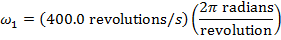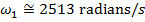The final angular velocity at time t1 = 5.0 s can be found by rearranging the angular acceleration formula: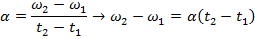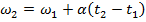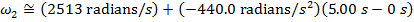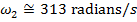The angular velocity after the magnetic brakes have been applied is approximately 313 radians/s. This is equivalent to 49.8 revolutions/s.